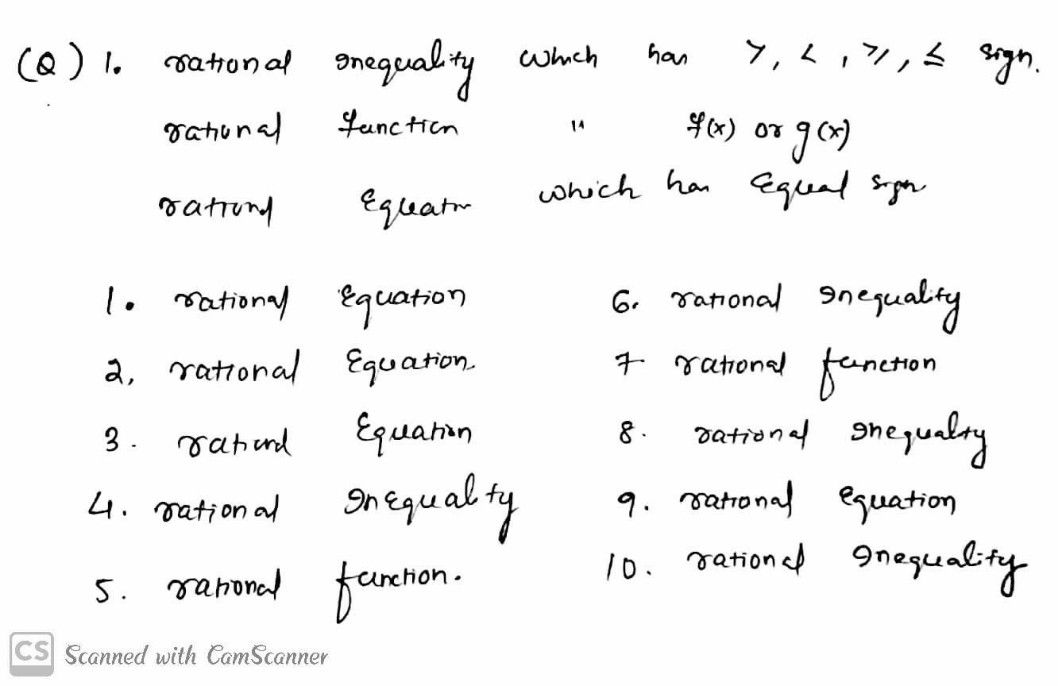Symbol
ProblemA. Determine whether the following is a rational equation, rational inequality or rational $5cote$ function. $⑤$ $\dfrac {x+1} {x-2}=\dfrac {x+2} {2x-1}$ 6. $\dfrac {2} {-3x+2}+4\leq 0$ 2. $\dfrac {1} {5x}-\dfrac {8} {x}=9$ 7. $g\left(x\right)=\dfrac {x^{2}-2x+1} {x-2}$ $y=\dfrac {2x+3} {x+1}$ $8$ $3x\geq \dfrac {8} {x+3}$ $4$ $\dfrac {3} {5x}>-4$ $9$ $\dfrac {x+4} {2x-5}=\dfrac {3} {x-2}$ $5$ $f\left(x\right)=\dfrac {x+3} {x^{2}-5}$ $10$ $\dfrac {2} {x}>\dfrac {6x} {2xs-1}$
10th-13th grade
Algebra
Search count: 126
SolutionQanda teacher - RRmamplease evaluate the answer and rate it